Courses

# Test: Ionic Bonds & Fajans' Rule

## 23 Questions MCQ Test Chemistry for JEE | Test: Ionic Bonds & Fajans' Rule

Description
This mock test of Test: Ionic Bonds & Fajans' Rule for JEE helps you for every JEE entrance exam. This contains 23 Multiple Choice Questions for JEE Test: Ionic Bonds & Fajans' Rule (mcq) to study with solutions a complete question bank. The solved questions answers in this Test: Ionic Bonds & Fajans' Rule quiz give you a good mix of easy questions and tough questions. JEE students definitely take this Test: Ionic Bonds & Fajans' Rule exercise for a better result in the exam. You can find other Test: Ionic Bonds & Fajans' Rule extra questions, long questions & short questions for JEE on EduRev as well by searching above.
QUESTION: 1

### Direction (Q. Nos. 1-16) This section contains 16 multiple choice questions. Each question has four choices (a), (b), (c) and (d), out of which ONLY ONE option is correct. Q. The correct statement for the molecule Csl-3 is

Solution: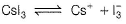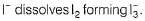Cs is electropositive element hence, it forms Cs+ ion. Hence, no covalent bonding.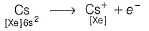Cs+ has stable inert gas configuration thus, Cs3+ is not formed.

QUESTION: 2

### Mg2+, 02- is formed when

Solution: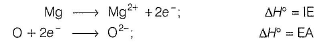Smaller the IE of Mg, easier the formation of Mg2+.
Greater the EA of O, easier the formation of O2-.

QUESTION: 3

### Solubility of KCI is maximum in

Solution:

KCI is an ionic compound. Force of attraction (F) between K+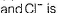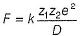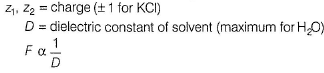Greater the value of D, smaller the value of F, hence larger the solubility.
Note D for CH3OCH3 is least out of given solvents, thus solubility is minimum.

QUESTION: 4

Lewis structure of MgCI2 and Al2O3 are

Solution:

Mg(1s2 2s2 2p63s2) is an electropositive element and Cl(1s2 2s2 2p6 3s2 3p5)is an electronegative element.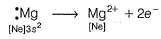Mg2+ attains stable inert gas configuration.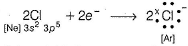Cl- also attains stable inert gas configuration.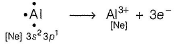Al3+ attains stable inert gas configuration.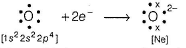O2- attains stable inert gas configuration.

QUESTION: 5

Lewis structure of the elements M and Z are shown below.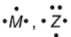Compound formed is

Solution: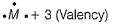(Three electrons extra than octet, it can donate three electrons)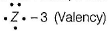(Three electrons short of octet, it can gain three electrons)
Thus, M3+ Z3-
Compound is MZ,

QUESTION: 6

Different kinds of bonds and interaction present within CuSO4 • 5H2O. They can be

I. σ-bond
II. π-bond
III. coordinate bond
IV. electrostatic force of attraction
V. H-bond due to dipole-dipole interaction
VI. H-bond due to ion-dipole interaction

Select the correct types of bonds/interactions.

Solution: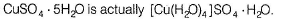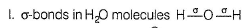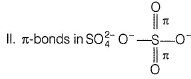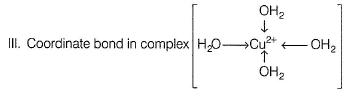IV. Electrostatic force of attraction between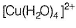and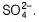V. H2O molecules joined together by dipole-dipole interaction.
VI. Outer H2O molecule joined toby ion-dipole interaction.

QUESTION: 7

Consider the following compounds

I. K4[Fe(CN)6]
II. NH4Cl
III. H2SO4
IV. [Ni (CO)4]

Q.
Ionic, covalent and coordinate bonds are present in

Solution: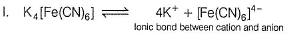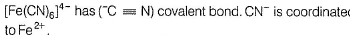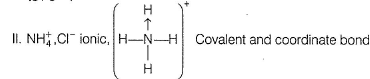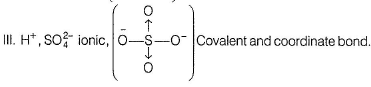QUESTION: 8

Out of the following, maximum covalent nature is in

Solution:

By Fajans rule,
Smaller the size of cation, larger the size of anion. Larger the charge on cation or anion, larger the polarising power and thus, greater the covalent nature.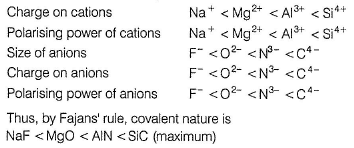QUESTION: 9

Arrange NaCI, MgCI2, AICI3, SiCI4 in increasing solubility in ether (non-polar solvent).

Solution:

Like dissolves like. Polar solvents (as H2O) dissolve polar compounds (ionic compounds) and non-polar solvents (as ether) dissolve non-polar compounds (covalent compounds).
By Fajans’ rule smaller the size of cation, larger the size of anion. Larger the charge on cation or anion then larger the polarising power and thus, larger the covalent nature and thus, solubility in non-polar solvents.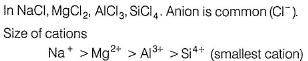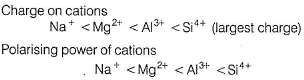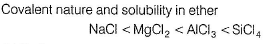QUESTION: 10

Covalent nature of NaF, Na2O and Na3N in the increasing order is

Solution:

By Fajans' rule,
Smaller the size of cation, larger the size of anion. Larger the charge on cation or anion, larger the polarising power and thus, greater the covalent nature.
NaF, Na2O, Na3N, Na+ is same.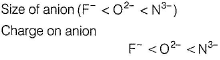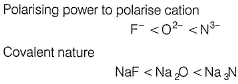QUESTION: 11

Solubility of CuS(l), ZnS (II) and Na2S (III) in water is in the order

Solution:

By Fajans' rule,
Smaller the size of cation, larger the size of anion.
Larger the charge ord-electrons present, then larger the polarising power of cation and larger the covalent nature and smaller the solubility in water.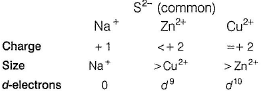Cu2+ due to shielding effect causes more screening than Zn2+.
Thus, polarising power of Na+ <Zn2+ < Cu2+ hence, ionic nature and solubility in water is in order
CuS < ZnS < Na2S

QUESTION: 12

Which pair is not in the correct order of lattice energy?

Solution:

Based on Fajans' rule,
Smaller the size of cation, larger the size of anion, larger the charge,
d-f-Aelectrons present then larger the polarising power, larger the covalent nature and larger the lattice energy.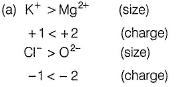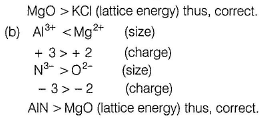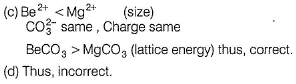QUESTION: 13

Most stable compound is

Solution:

By Fajans’ rule,
Smaller the size of cation, larger the size of anion, larger the charge, then larger the polarising power, larger the covalent nature, means low melting point.
Smaller the ionic nature, means low melting point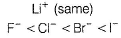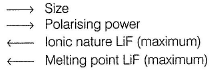Hence, most stable is LiF.

QUESTION: 14

Out of the following ions, which pair wil make the compound most covalent?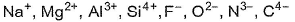Solution:

By Fajans’ rule, smaller the size of cation, larger the size of anion, larger the charge on cation or anion,
d- and f-electrons present on the ion then greater the polarising power of the ion to polarise the other ion, thus larger the covalent nature.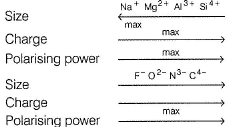Thus, com pound with Si4+ (smallest cation) and C4- (largest anion) will be most covalent.

QUESTION: 15

Aqueous solution of a mixture contains LiCI, CuCI, NaCI and AICI3. This is shaken with ether. What is/are left in water?

Solution:

Polarising power of Li+ > Na+ and AI3+ > Na+
Thus, LiCI and AICI3 have covalent nature and extracted into ether.
CuCI due to d-electrons has covalent nature. (Polarising power of Cu+ > Na+).
Thus, only NaCI (ionic) remains in water.

QUESTION: 16

Select the correct statement(s).

Solution:

Cu+ = [Ar] 3d10, Cu+ has inner 18-electrons shell. Shielding effect is decreased. Thus, electronegativity is increased. This results in greater polarising power of Cu+ .
Hence, CuCI is covalent.

*Multiple options can be correct
QUESTION: 17

Direction (Q. Nos. 17-20) This section contains 4 multiple choice questions. Each question has four choices (a), (b), (c) and (d), out of which ONE or  MORE THANT ONE  is correct.

Q. Which of the following can be calculated based on Born-Haber cycle of formation of a lattice A+B- (s) from A(s) and 6 (g) ?

Solution:

Born Haber cycle involves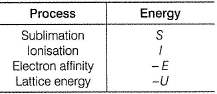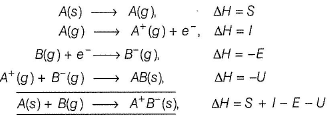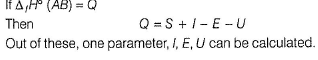*Multiple options can be correct
QUESTION: 18

Which of the following Lewis acid-Lewis base interactions are associated with the expansion of octet?

Solution:

(a) In SbF5 (expansion of octet from 5 to 10 and further to 12).
(b) NH3, BF3 both have complete octet. NH3 is electron rich, donates lone pair to BF3.
(c) AICI3 is electron deficient, accepts lone pair from Cl-.
(d) In SF4, expansion of octet from 8 to 10 and further to 12.

*Multiple options can be correct
QUESTION: 19

An ionic compound A+B- is most like to be formed from A and B when

Solution:

A → A+ + e- is favourable since, IE of A is low.
B + e- → B- is favourable since, EA of B is high.
Thus, A+B- is favoured by low IE of A and high EA of B.

QUESTION: 20

Consider following types of bonds.

I. Ionic bond
II. Covalent σ - bond
III. Coordinate bond
IV. Covalent π - bond

Q.
All these bonds are present in

Solution: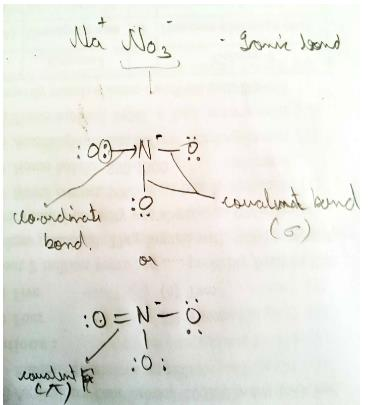QUESTION: 21

Direction (Q. Nos. 21-22) This section contains  a paragraph, wach describing theory, experiments, data etc. three Questions related to paragraph have been given.Each question have only one correct answer among the four given options (a),(b),(c),(d)

A+B- (ionic) is formed in the following steps from gaseous atoms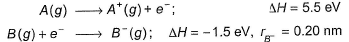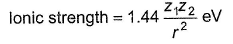Q.
How much energy is required to transfer one electron from A to B?

Solution: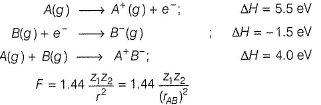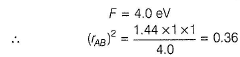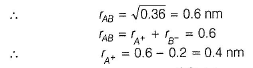QUESTION: 22

A+B- (ionic) is formed in the following steps from gaseous atomsQ. What is the maximum size of Athat would lend itself for the formation of an energetically stable bond?

Solution:*Answer can only contain numeric values
QUESTION: 23

Direction (Q. Nos. 23) This section contains 1 question. when worked out will result in an integerfrom  to 9 (both inclusive)

Q.
An ionic bond is established between a positive ion A+ and negative ion B- . How many times strength of the ionic bond is affected by doubling the charge on A+ and making the radius halved?

Solution: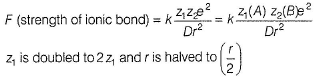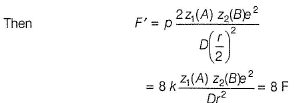Thus, ionic strength becomes 8 times.